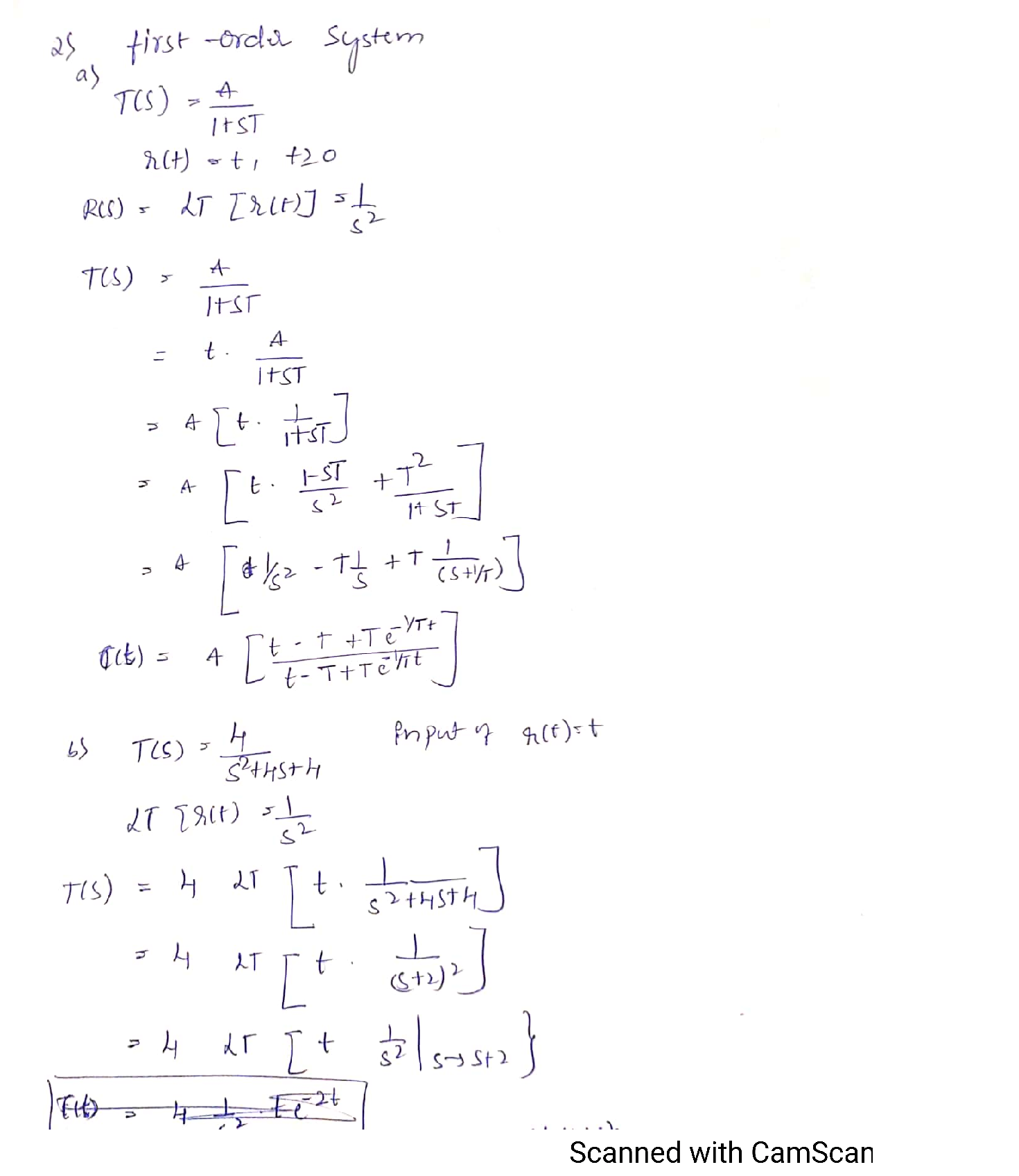Question

Control System

(a) The first-order system has the transfer function

T(s)=\frac{A}{1+s T}

Find the system output response in time domain when a unit ramp signal, r(t)=t, is applied to its input.

(b) The transfer function of a second-order system is:

T(s)=\frac{4}{s^{2}+4 s+4}

Find the system output response in time domain when a unit ramp signal, r(t)=t, is applied to its input.

\text { (Note: The inverse Laplace transform of } F(s)=\frac{1}{(s+a)^{2}} \text { is } f(t)=t \cdot e^{-a t} \text {.) }Verified### Question 45498Control System

2. For the system shown below, find the output c(t) if the input r(t) is a unitstep. For full credit, transfer function should be computed correctly, C(s)should be computed correctly, and c(t) should be computed correctly.Partial credit may be earned.

### Question 45497Control System

1. Reduce the block diagram shown below to a single block,T(s) = C(s)/R(s). For full credit, all loops should be correctly reduced.Partial credit may be earned for correctly reducing some loops.

### Question 44984Control System

a) Knowing the format of state space representation:
\dot{x}=A x+B u
y=C x+D u
If a second order system is modeled in a state space representation, what will be the dimensions of Aand B matrix?
b) Consider a system modeled via the third order differential equation:
\dddot{x}+3 \ddot{x}+4 \dot{x}+x=5 \dot{u}+u
Where, x(t) is displacement, u(t) is force. Develop a state space representation for the system. The desired output is all state variables.

### Question 44983Control System

Q1) Given the following block diagram system.
a) Reduce the block diagram to a single transfer function.
b) Plot the root locus of the system. Explain the plot.

### Question 44964Control System

O Which of the following statements is incorrect?
i. Root-locus is symmetric about x-axis.
ii. Root-locus starts at open-loop poles and ends at open-loop zeros.
iii. Root-locus graph tracks the closed-loop poles as the gain varies.
iv. None.

### Question 44963Control System

) If the open-loop transfer function is given by
\frac{K(s+2)}{s^{4}+2 s+2}
how many open-loop zeros are at infinity?
i. 1ii. 3iii. 4iv. 0

### Question 44962Control System

) If KG(s)H(s) represents the open-loop transfer function of a system, then thegain K and the closed-loop poles of the system satisfy which equation?
i. KG(s)H(s) =1
ii. KG(s)H(s) = -1
ii. KG(s)Н (s) — 0
iv. KG(s) = H(s)

### Question 44961Control System

If the static error constant K.100, then the stead-state errors for step and ramp inputs are
\text { i. } e_{\text {rtep }}(\infty)=0, e_{\text {ramp }}(\infty)=0
\text { ii. } e_{\text {step }}(\infty)=0, e_{\text {ramp }}(\infty)=\infty
\text { iii. } e_{\text {step }}(\infty)=\infty, e_{\text {ramp }}(\infty)=0
\text { iv. } e_{\text {step }}(\infty)=\infty, e_{\text {ramp }}(\infty)=\infty

### Question 44960Control System

) In the unity feedback system in Figure 1, at least how many poles of G(s) mustbe at the origin for the steady-state error due to the ramp input is zero?
i. 1ii. 2iii. 3iv. 4

### Question 44959Control System

(5 pts.) Sketch the root locus (rough sketch) for the following problems, and for each problem find the range of K where the system is stable.

### Submit query

Getting answers to your urgent problems is simple. Submit your query in the given box and get answers Instantly.

### Submit a new QuerySuccess

Assignment is successfully created